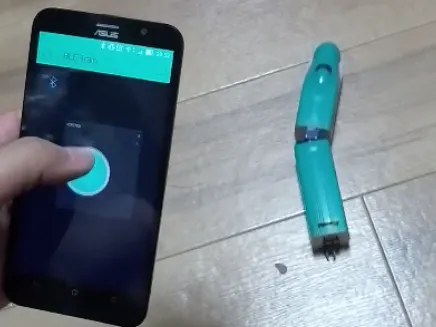Published

# BLE Toy Train Controlled by Blynk

I made train controlled by Blynk and BLE board. I can easily using Blynk!!

BeginnerFull instructions provided2 hours1,709## Things used in this project

### Hardware components

 RedBear BLE Nano
×1

### Software apps and online servicesArduino IDEBlynk

## Code

### BleTrain_Blynk01.ino

Arduino
```#define BLYNK_PRINT Serial

#include <BlynkSimpleRedBearLab_BLE_Nano.h>
#include <BLE_API.h>
#include <Wire.h>
#include <Servo.h>

Servo myservo;
const int DRV8830 = 0x64;

int power = 0;
float angle = 0;
int rot = 0;

int Speed = 0;
int angleServo_ini = 35;
int angleServo = angleServo_ini;

// You should get Auth Token in the Blynk App.
char auth[] = "YourAuthToken";

uint8_t device_name[] = "Blynk";

//motor driver I2C control
//Reference
//http://makers-with-myson.blog.so-net.ne.jp/2014-05-15
void writeMotorResister(byte vset, byte data1){
int vdata = vset << 2 | data1;
Wire.beginTransmission(DRV8830);
Wire.write(0x00);
Wire.write(vdata);
Wire.endTransmission(true);
}

void setup() {
Wire.begin(D3, D2, 400000L);
Serial.begin(250000);
writeMotorResister(0x00, 0x00);
myservo.attach(D4);
myservo.write(angleServo);

delay(100);

ble.init();

device_name, sizeof(device_name) - 1);

ble.gap().setDeviceName(device_name);
ble.gap().setTxPower(4);

Blynk.begin(auth);

Serial.println("Waiting for connections...");
}

//Joystick value
BLYNK_WRITE(V1) {
float x = param.asInt() - 128.0;
float y = param.asInt() - 128.0;

//motor speed
power = sqrt(x*x + y*y);
if(power > 128){
power = 128;
}
Speed = map(power, 0, 128, 5, 19);

//servo angle
angle = atan(y/x) * 180.0 / PI;
if(x < 0 && y > 0){
angle = angle * -1;
}else if(x > 0 && y >= 0){
angle = 180.0 - angle;
}else if(x >= 0 && y < 0){
angle = 180.0 + angle;
}else if(x == 0 && y == 0 || power >= 0 && power < 57){
angle = 90;
}
angleServo = map(angle, 180, 0, 0, angleServo_ini * 2);

//motor rotation
if(y >= 0){
rot = 2; //Forward
} else {
rot = 1; //Reverse
}

if (power == 0){
writeMotorResister(0x00, 0x00);
}else {
writeMotorResister((byte)Speed, (byte)rot);
}
myservo.write(angleServo);

Serial.print("x: ");
Serial.print(x);
Serial.print("  y: ");
Serial.print(y);
Serial.print("  power: ");
Serial.print(power);
Serial.print("  Speed: ");
Serial.print(Speed);
Serial.print("  angleServo: ");
Serial.print(angleServo);
Serial.print("  rot: ");
Serial.println(rot);
}

void loop() {
Blynk.run();
}
```